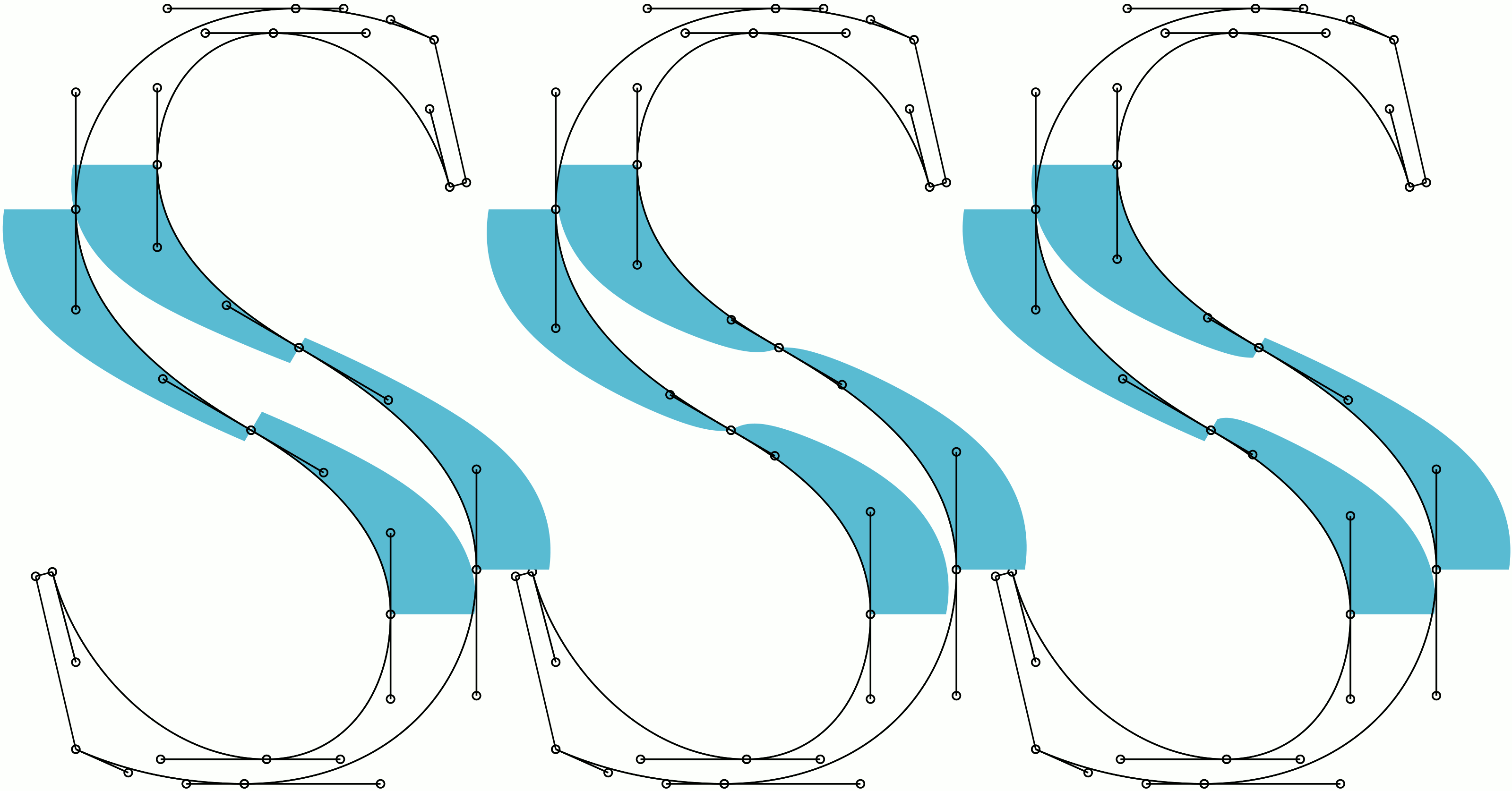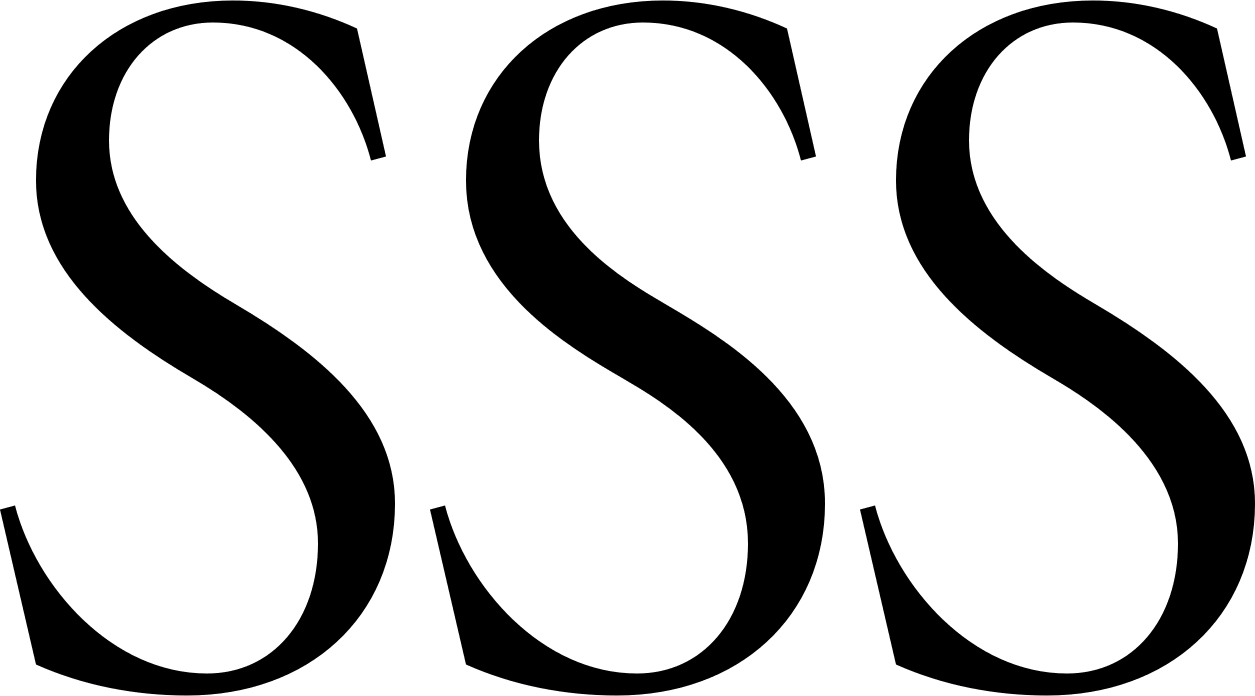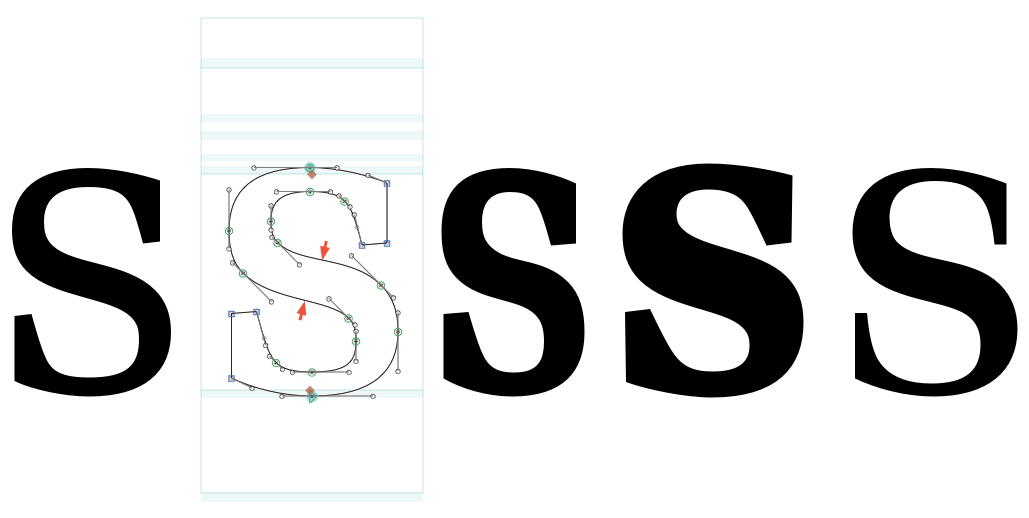#### Howdy, Stranger!

It looks like you're new here. If you want to get involved, click one of these buttons!

# Handles Near Inflection Node

I did quite some mathematical investigation in "harmonizing" cubic Bézier curves. However, I am not sure how to handle the problem with handles near an inflection node. The following picture shall be an example of this problem (the blue areas are curvature combs):
The left S is a draft. The middle and the right S are possible improvements of the segments near the inflection nodes.The problem with the draft (left) is, that the curvature changes abruptly. Now it would be mathematically advisable to change the handles such that the curvature in the inflection is 0 (mid). However, this means that the handles attached to the neighbour nodes have to reach exactely the intersection point of the node tangents - and this is critical for two reasons:
1. Rounding issue: After rounding, the handles may exceed the tangent triangle which means that an additional inflection point occurs on the segment.
2. Interpolation issue: Even if rounding is handled correctly, the same problem may occur after interpolation
Of course, one could remove the inflection node completely but this gives less control over the curve (less degree of freedom).
The S to the right changed the handles such that the curvature on both sides is the minimum of the curvature before on both sides. This is the best I could think of but it is an arbitrary choice. An other arbitrary choice could be to choose the technique in the middle but rescale the handles by e.g. 90%.

What choice would you recommend? («Use your eyes» is not a useful advice for me, because I need this for an algorithm).Tagged: